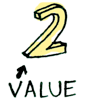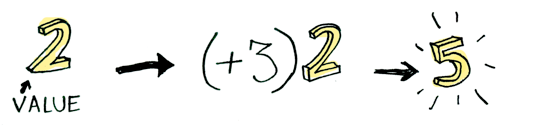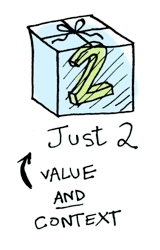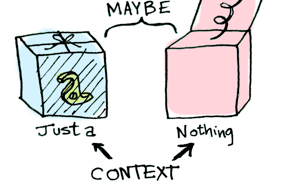Swift:

enum Optional<T> {
case None
case Some(T)
}

data Maybe a = Nothing | Just a

Optional和Maybe类似两个盒子，其中包裹着两个不同的值函数：无(None)和有(Some)。

Functor函子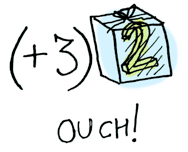func plusThree(addend: Int) -> Int {
}

Optional.Some(2).map(plusThree)
// => .Some(5)

> fmap (+3) (Just 2)
Just 5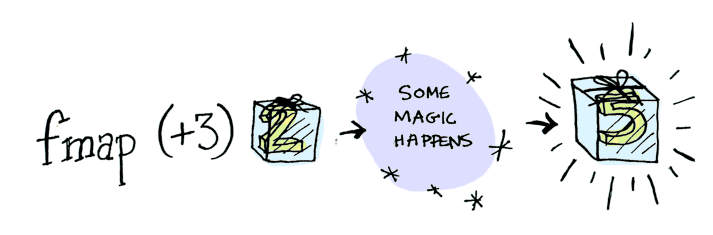fmap告诉我们它已经成功完成，但是它是如何应用函数到盒子里的值呢？

函子到底是什么?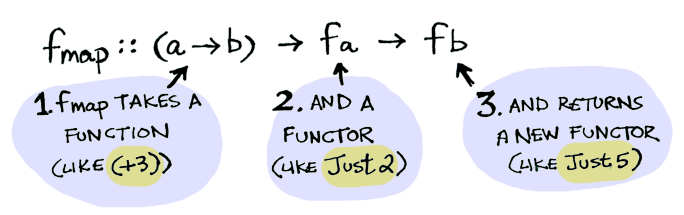Optional.Some(2).map { \$0 + 3 }
// => .Some(5)

func map<U>(f: T -> U) -> U? {
swifth self {
case .Some(let x): return f(x)
case .None: return .None
}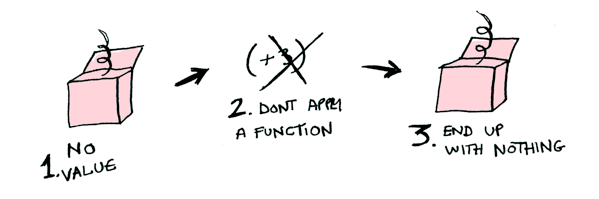Optional.None.map { \$0 + 3 }
// => .None

map或fmap知道如果你应用函数到None，你同样得到的还是无None，这里map或fmap是不是像禅一样呢？

let post = Post.findByID(1)
if post != nil {
return post.title
} else {
return nil
}

findPost(1).map(getPostTitle)

getPostTitle <\$> (findPost 1)

Swift代码如下：

infix operator <^> { associativity left }

func <^><T, U>(f: T -> U, a: T?) -> U? {
return a.map(f)
}
getPostTitle <^> findPost(1)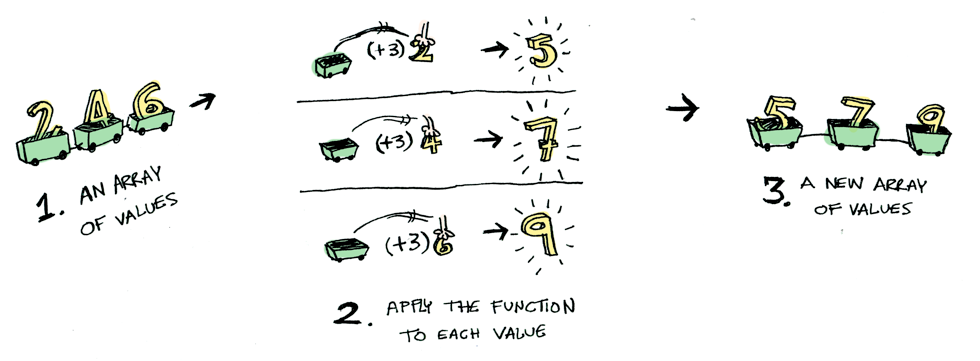instance Functor [] where fmap = map

fmap (+3) (+1)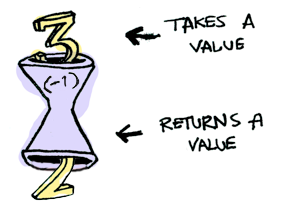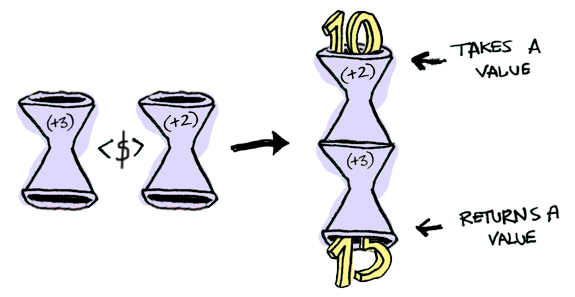> import Control.Applicative
> let foo = fmap (+3) (+2)
> foo 10
15

instance Functor ((->) r) where fmap f g = f . g

Swift将一个函数应用到另外一个函数的代码如下：

typealias IntFunction = Int -> Int

func map(f: IntFunction, _ g: IntFunction) -> IntFunction {
return { x in f(g(x)) }
}

let foo = map({ \$0 + 2 }, { \$0 + 3 })
foo(10)
// => 15